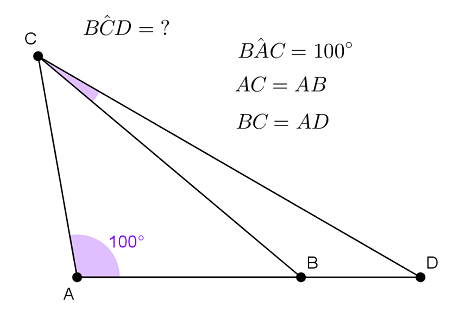# Tiny angleIn triangle $CAD$ we have $\hat { CAD } = 100°$. Point $B$ in on $AD$ such that $AC = AB$ and $BC = AD$. Find $\hat { BCD }$ in degrees.

×